How do you decide if two biological variables are related? There is a vast amount of literature on the topic, but chances are one of the first tools you reach for is a correlation metric. They're straightforward and cheap to calculate, which is critical when checking for over a billion possible relationships as we do in DepMap. But which correlation metric? There's the classic Pearson correlation:

$\rho(x, y) = \frac{x - \mu_x}{\sigma_x} \cdot \frac{y - \mu_y}{\sigma_y}$

and the Spearman correlation, which is just the correlation of the rankings of samples in the two variables:

$r(x, y) = \rho(\mathrm{rank}(x), \mathrm{rank}(y))$

Perhaps you've heard that Pearson correlation coefficients are only suitable for linear relationships. Many biological relationships can't be linear because one of the variables is constrained to some domain. For example, a gene can't have fewer than 0 transcripts, and a drug can't kill more than 100% of cells. The relationship between gene expression and drug response might be monotonic, but probably isn't linear. A natural correlation measure for nonlinear monotonic, nonlinear relationships is Spearman correlation, and indeed it's been commonly used in papers such as this controversial comparison of drug datasets. In addition to being nonlinear, Spearman correlations are more robust to outliers than Pearson.

So why do we primarily use Pearson instead of Spearman correlation coefficients in the DepMap Portal?

### A Toy Example

Let's consider the ideal case for a Spearman correlation, in an experiment with no noise, a normally distributed independent variable A, and a variable B exactly equal to some logistic function of A:

import numpy as np
import pandas as pd
import seaborn as sns
from matplotlib import pyplot as plt
from scipy.stats import pearsonr, spearmanr

def simpleaxis(ax=None):
'''removes the top and right boundaries from pyplot plots for aesthetic reasons'''
if ax is None:
ax = plt.gca()
ax.spines['top'].set_visible(False)
ax.spines['right'].set_visible(False)
ax.get_xaxis().tick_bottom()
ax.get_yaxis().tick_left()

A = np.random.normal(size=1000, scale=3)
B = np.exp(-A)/(1+np.exp(-A))

plt.scatter(A, B, s=10, alpha=.4, edgecolor='navy')
plt.xlabel('A')
plt.ylabel('B')
simpleaxis()

plt.title("No Noise")

print("Pearson correlation: %1.2f" % pearsonr(A, B))

print("Spearman correlation: %1.2f" % spearmanr(A, B))


Pearson correlation: -0.94
Spearman correlation: -1.00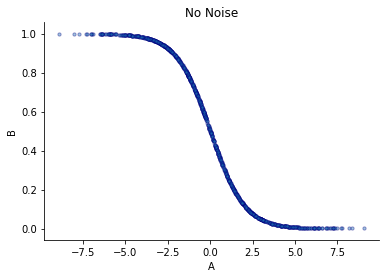In this case we know the variables are deterministically related, so a higher correlation measure indicates better performance. The Spearman coefficient is better capturing the fact that this is an exact relationship than Pearson does, although not by much. Of course, real data is not noise-free. What happens if the relationship becomes noisier?

vertical_noise_scale = .1
vertical_noise = np.random.normal(size=len(A))
horizontal_noise_scale = .1
horizontal_noise = np.random.normal(size=len(A))
A2 = A + horizontal_noise_scale * horizontal_noise
B2 = B + vertical_noise_scale * vertical_noise

plt.scatter(A2, B2, s=10, alpha=.4, edgecolor='navy')
plt.xlabel('A')
plt.ylabel('B')
simpleaxis()

plt.title("With Noise")

print("Pearson correlation: %1.2f" % pearsonr(A2, B2))

print("Spearman correlation: %1.2f" % spearmanr(A2, B2))

Pearson correlation: -0.91
Spearman correlation: -0.95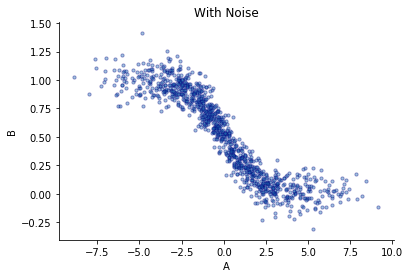We still see that Spearman correlation better captures the relationship than Pearson. But in addition to noise, biological variables are also often non-normally distributed. In fact, when we look for interesting gene targets for therapeutic intervention in genetic perturbation screens, we often look for explicitly non-normal distributions. What happens if A has a dominant “non-responder class” centered at positive values, and a minority “responder” class in its left tail?

class_fraction= .95
A3 = np.random.normal(size=int(1000*class_fraction), scale=1, loc=4)
A3 = np.concatenate([A3, np.random.normal(size=1000-len(A3), scale=1, loc=-4)])
B3 = np.exp(-A3)/(1+np.exp(-A3))

A3 += horizontal_noise_scale * horizontal_noise
B3 += vertical_noise_scale * vertical_noise

plt.scatter(A3, B3, s=10, alpha=.4, edgecolor='navy')
plt.xlabel('A')
plt.ylabel('B')
simpleaxis()

plt.title("With Class Imbalance")

print("Pearson correlation: %1.2f" % pearsonr(A3, B3))

print("Spearman correlation: %1.2f" % spearmanr(A3, B3))

Pearson correlation: -0.83
Spearman correlation: -0.35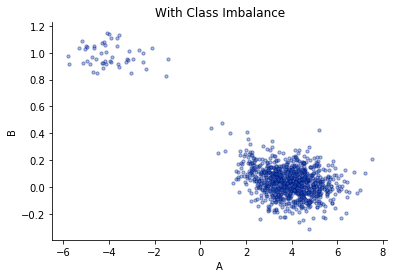Although it's visually obvious that these variables are still strongly related, now Spearman correlation registers only a weak relationship. If we plot the rankings of samples in A and B, the reason for this is clear:

plt.scatter(np.argsort(A3), np.argsort(-B3), s=10, alpha=.4, edgecolor='navy')
plt.xlabel('A Ranking of Sample')
plt.ylabel('B Ranking of Sample (Reversed)')
simpleaxis()

plt.title("Ranking of A vs Ranking of B")

Text(0.5, 1.0, 'Ranking of A vs Ranking of B')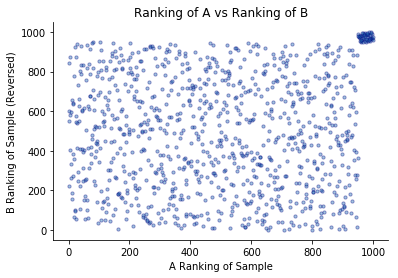Spearman relies solely on rank ordering of the data. The high number of randomly distributed values in the large “nonresponder” group in the bottom left thwarts this metric.

Does this example seem contrived? Take a look at ERBB2 gene dependency vs expression for a real-world case where Pearson correlation better captures the (known) relationship than Spearman. Examples like these are surprisingly common among compelling cancer dependencies.

### Word of Caution

Of course, Pearson correlations come with their own failure modes. A single shared outlier between two variables can be enough to drive a Pearson correlation that seems highly significant until you examine the scatter plot:

A4 = np.random.normal(size=1000, scale=3)
B4 = np.random.normal(size=1000, scale=3)

A4 = B4 = 50

plt.scatter(A4, B4)
plt.title('Single Outlier')
print("Pearson correlation: %1.2f" % pearsonr(A4, B4))

print("Spearman correlation: %1.2f" % spearmanr(A4, B4))

Pearson correlation: 0.25
Spearman correlation: 0.03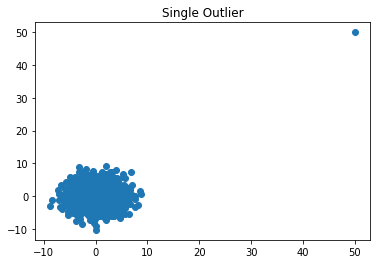When testing many associations, it's important to use filters to catch cases like these. For example, you might want to z-score one of the variables and throw out the most extreme point. However, once more than one point belongs to the minority class, our confidence in the meaning of a strong Pearson correlation grows rapidly.

## Overall, Pearson just seems to work better for DepMap data

We usually find that it's easier to control for a well-understood cause of false positives from Pearson correlations with single outliers than to recover the false negatives that occur with Spearman correlation for unbalanced classes. This and computational simplicity explain why we've settled on Pearson correlation as the first-line method of testing associations in the Dependency Map.